• ## 卡尔曼滤波预测

热门讨论 2012-05-10 11:13:59
卡尔曼滤波预测，从数据的加载，到卡尔曼滤波作图，全有
•研究论文
• 基于卡尔曼滤波预测结合矢量场矩形算法的移动机器人避障研究研究论文
• 卡尔曼滤波预测轨迹，非常有用，从纳什均衡问题的求解现状和粒子群算法的发展出发，对纳什均衡问题进行分析，并且使用粒子群算法模拟纳什均衡的博弈过程。最终使用Matlab软件实现，最后简单的改进了一下算法的初值和...
• 5 Robotics: Estimation and Learning GMM预测+卡尔曼滤波WEEK 1Programming Assignment: Color Learning and Target Detection 首先这个系列的第一个单元是空中机器人，第二单元运动规划，第三单元感知（相机方面）...
5 Robotics: Estimation and Learning GMM预测+卡尔曼滤波预测WEEK 1Programming Assignment: Color Learning and Target DetectionWEEK 2Kalman Filter Tracking
首先这个系列的第一个单元是空中机器人，第二单元运动规划，第三单元感知（相机方面）
以下博客如下：
1 Robotics: Aerial Robotics 第1+2周 课程学习记录及课后习题解答
1 Robotics: Aerial Robotics 第3+4周 课程学习记录及课后习题解答
2 Robotics: Computational Motion Planning 第1周（内含Dijkstra 和 A*  MATLAB代码手把手教学）课后习题解答
2 Robotics: Computational Motion Planning 第2+3+4周 课后习题解答
3 Robotics: Mobility 课程学习记录及课后习题解答
4 Robotics: Perception 课程学习记录及课后习题解答

此课程在Coursera需要科学上网才能观看，放一下B站和Coursera的课程链接

UP主 博主自己做的字幕版本 这个单元会全部更新完（持续更新）
Coursera的链接介绍
此文仅为听课记录以及做题思考，有所错误的地方或是好的想法欢迎评论区交流。（点个赞就更好了，鼓励一下~）

3.5：听完第一周，我觉得这个单元是这个系列里的最好了吧，第二是运动规划（主要是作业非常引人深思），第三是空中机器人；后面过程中，我讲吧，这周主要是高斯模型和GMM，一起预测小球位置什么的，具体可以看我翻译上传的，（这周超爽只有编程作业，哈哈哈哈哈哈），第一周很有意思，有几个点可能会不知道怎么办，那么开始了。写完啦~ 下一周慢慢来~ 这周编程over了
3.6：这卡尔曼滤波十分有意思，但是这个系列的课程讲的真的不是很好，建议如果想真的明白一点，走讨论区的链接（贼仔细）Youtube Michel van Biezen The Kalman Filter（不想看视频/看不了的可以直接看我笔记，我觉得我记得十分详细… ），当然编程题目还是要做的啦，那么开始啦

WEEK 1
Programming Assignment: Color Learning and Target Detection
这一切的前提，麻烦点击b站视频先看完这周的视频，我相信你会对GMM有个完整的了解同时用EM方法计算等，接下来就开始作业了。
作业的pdf其实说的清楚顺序，但是有些详细的没说清，
1.打开example_rgb.m然后，这里不是说你运行，标完点就over了（要训练多边形），后面是要写个高斯的，计算$\mu$和$\sigma$的
这里如果已经运行完，计算方差的时候可能会报错：
MTIMES (*) 不完全支持整数类。至少一个参数必须为标量。

出错 cov (line 155)
c = (xc' * xc) ./ denom;

出错 example_rgb (line 57)
sigma(i) = cov(Samples(:,i));

这是因为你的Samples是unit8的，所以这个有两种解决方法：1.不用RGB来作为高斯的训练，而是另一种HSV（H色调，S饱和度，V明度），因为这个是double类型，而且和RGB一样，也是3个，3维训练的。2.如果你好不容易已经标完了多边形，有了RGB的样本点了，那么还有另一种方法就是！强制转换double(Sample(:,1))即可。具体代码如下：（添加在example_rgb.m后面运行部分即可）
%%%%%%%%%%%%%%%%%%%%%%%%%%%%%%%%%%%%%%%%%
% [IMPORTANT]
%
% Now choose you model type and estimate the parameters (mu and Sigma) from
% the sample data.
%%
D=3; %确定维度
mu=zeros(1,D);
sigma=zeros(1,D);
for i=1:D
mu(i)=mean(Samples(:,i));
sigma(i)=cov(double(Samples(:,i)));
end
mu%显示一下mu
sigma%显示一下sigma

这里我们需要记录mu和sigma的值也就是显示出来的：
mu =

148.4140  144.0171   62.4389

sigma =

208.4049  133.1187  375.4475

这个有什么用呢？emm 如果你不知道 那证明你得回去看视频课（反正能1.5倍速快速回顾一下吧） -> 没错 初始化！（接下来是一个个讲的如果觉得废话了，可以跳转到完整代码区哈~）
第一部分代码如下：
%%%%%%%%%%%%%%%%%%%%%%%%%%%%%%%%%%%%%%%%%%
% Hard code your learned model parameters here
%
mu = [148.4140  144.0171   62.4389];
sigma = [208.4049  133.1187  375.4475];
thre =  0.2e-5;

然后大家可能会问了：初始化了mu和sigma还有个thre你没初始化，为什么有值呀？
那个嘛… 一开始我也不知道啥 随便给了个0.0000001然后去讨论区，助教的一句话点醒了
特此复制过来：
b. To set threshold you have to visualize your probability matrix (probability of all pixels in image based on your model) .Use surf or histogram for visualizing it and then example_test.m to see how your parameters are doing. 完整链接跳转 点击此处
也就是说如果要设置阈值，你先得看看你的可能性函数长啥样
那么：让我们开始吧，第二部分代码如下：
%%%%%%%%%%%%%%%%%%%%%%%%%%%%%%%%%%%%%%%%%%
% Find ball-color pixels using your model
%
[mrows,mcols,D]=size(I);
prob_den=[];
for i=1:mrows
for j=1:mcols
prob_den(i,j)=mvnpdf(double([I(i,j,1),I(i,j,2),I(i,j,3)]),mu,sigma);
if prob_den(i,j)>thre
end
end
end
figure,surf(prob_den);

很多人可能问了,mvnpdf是啥？emm 你看看我写的啥，是不是有点像求那个点的高斯函数值？是的！大家help一下就能看明白了，特此把MATLAB的说明也复制过来吧：
y = mvnpdf(X,mu,sigma) returns pdf values of points in X, where sigma determines the covariance of each associated multivariate normal distribution.
Specify [] for mu to use its default value of zero when you want to specify only sigma.
最后的figure,surf(prob_den)就是我画可能性的图了，那么图开始了：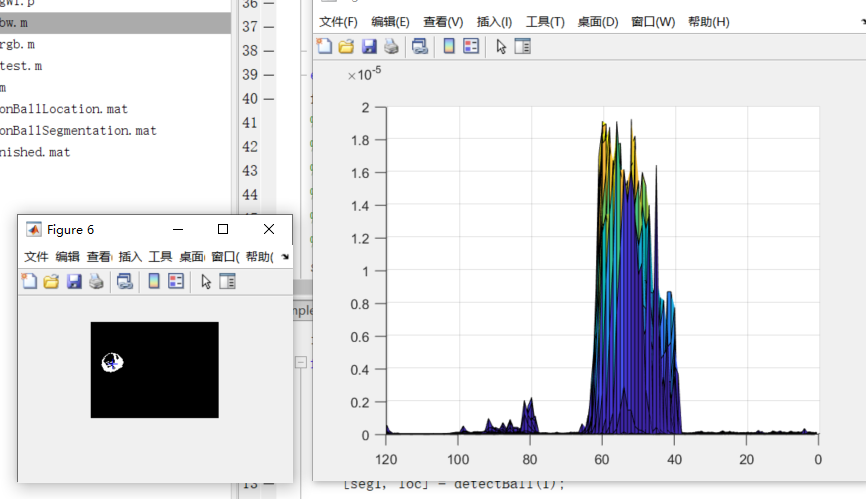这里能看到什么？看到了，emm 大概给个0.2*10-5球的位置差不多能被划分，
接下来我们看看其他球照片：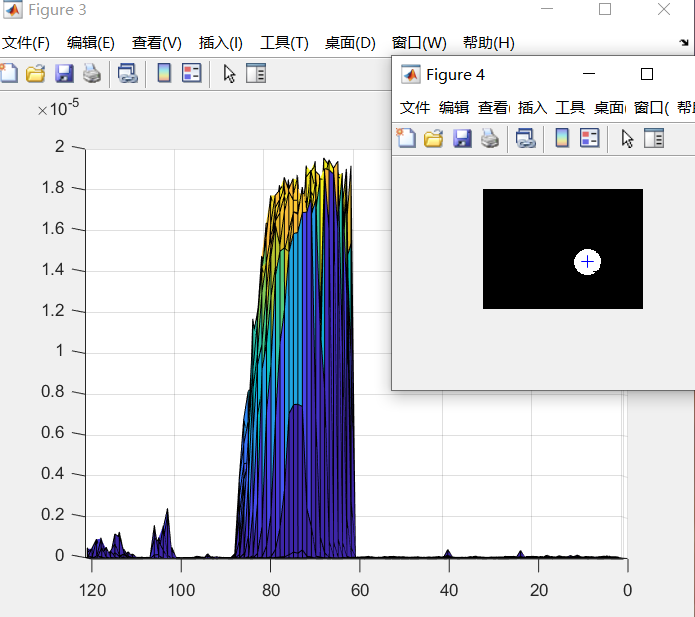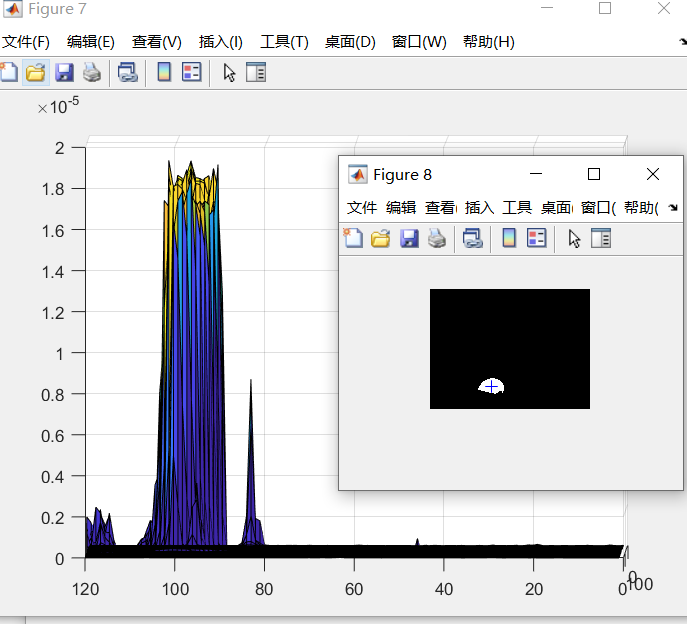第三张照片可能更突然一点，也就是0.2似乎小了，应该放到0.8比较好，其实… 不太好，因为就这一个一点点有，所以，以此确定咱们的thre=0.2e-5（这个是MATLAB的科学计数法哈）
完成初始化和第二部分基本就over了，因为后面是输出数的格式而已，文档也说了，请参考example_bw进行改写即可。
所以这个编程作业的主要难点在：1.初始化！很多人不知道怎么去看mu,sigma,thre怎么初始化的，2.两个for循环那段可能不太清楚怎么写（其实应该清楚，只是需要一个指引罢了）
那么我就贴 完整代码（在这里） detectBall.m：
% Robotics: Estimation and Learning
% WEEK 1
%
% Complete this function following the instruction.
function [segI, loc] = detectBall(I)
% function [segI, loc] = detectBall(I)
%
% INPUT
% I       120x160x3 numerial array
%
% OUTPUT
% segI    120x160 numeric array
% loc     1x2 or 2x1 numeric array

%%%%%%%%%%%%%%%%%%%%%%%%%%%%%%%%%%%%%%%%%%
% Hard code your learned model parameters here
%
mu = [148.4140  144.0171   62.4389];
sigma = [208.4049  133.1187  375.4475];
thre =  0.2e-5;

%%%%%%%%%%%%%%%%%%%%%%%%%%%%%%%%%%%%%%%%%%
% Find ball-color pixels using your model
%
[mrows,mcols,D]=size(I);
prob_den=[];
for i=1:mrows
for j=1:mcols
prob_den(i,j)=mvnpdf(double([I(i,j,1),I(i,j,2),I(i,j,3)]),mu,sigma);
if prob_den(i,j)>thre
end
end
end
figure,surf(prob_den);
%%%%%%%%%%%%%%%%%%%%%%%%%%%%%%%%%%%%%%%%%%
% Do more processing to segment out the right cluster of pixels.
% You may use the following functions.
%   bwconncomp
%   regionprops
% Please see example_bw.m if you need an example code.
segI=false(mrows,mcols);
numPixels = cellfun(@numel,CC.PixelIdxList);
[biggest,idx] = max(numPixels);
%%%%%%%%%%%%%%%%%%%%%%%%%%%%%%%%%%%%%%%%%%
% Compute the location of the ball center
%

segI(CC.PixelIdxList{idx}) = true;
S = regionprops(CC,'Centroid');
loc = S(idx).Centroid;
%
% Note: In this assigment, the center of the segmented ball area will be considered for grading.
% (You don't need to consider the whole ball shape if the ball is occluded.)
end


虽然我的这个可能后面划分也有点问题，但是整个划分的范围还是对的，所以提交也得了满分~ 有其他想法的也可以一起交流，顺便点个赞，谢谢各位。
WEEK 2
Kalman Filter Tracking
emm 还是那句话 最好先看视频（尽管这个系列讲的不好，但是有个人讲的很好呀 -> Youtube Michel van Biezen The Kalman Filter）
放听课笔记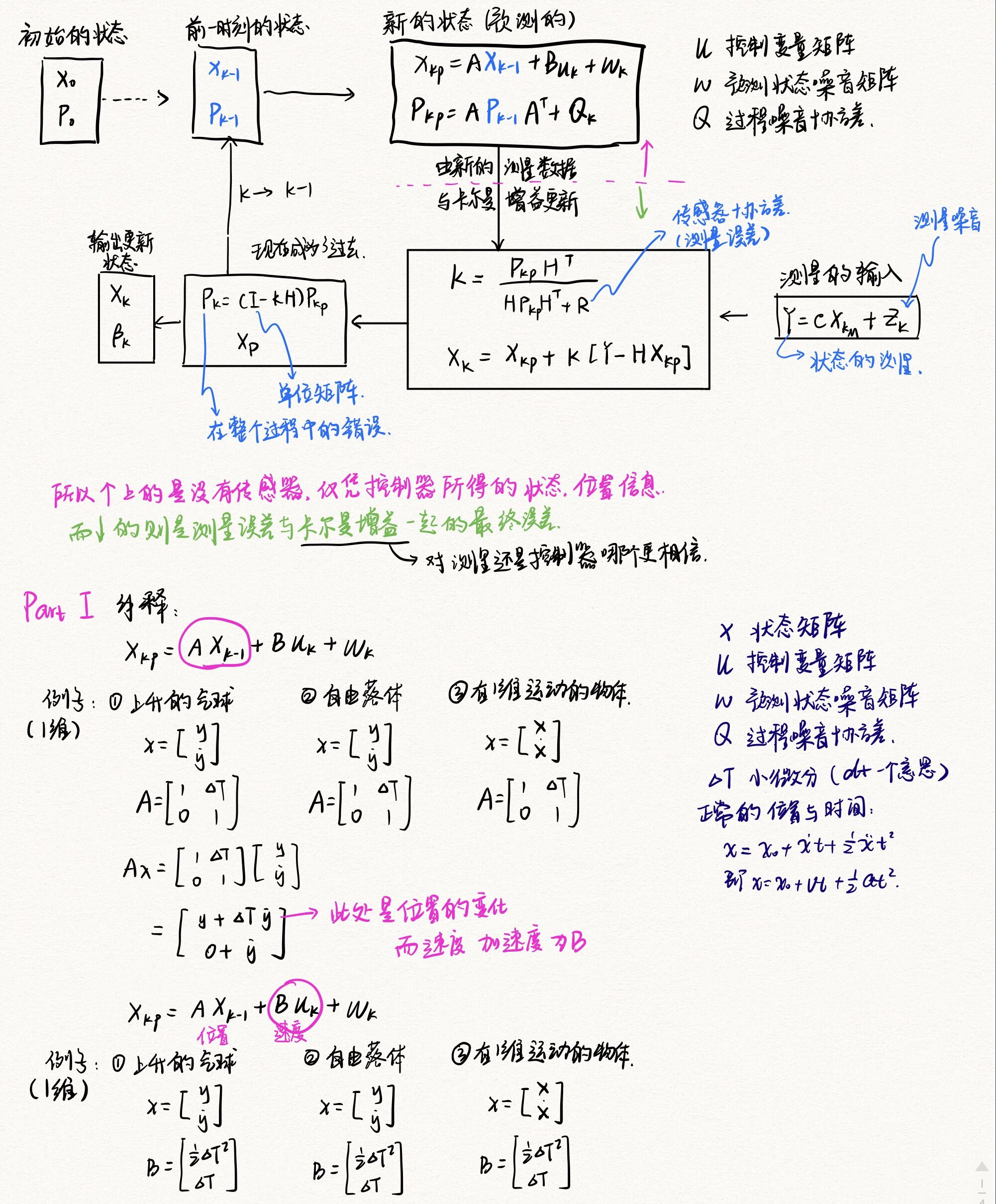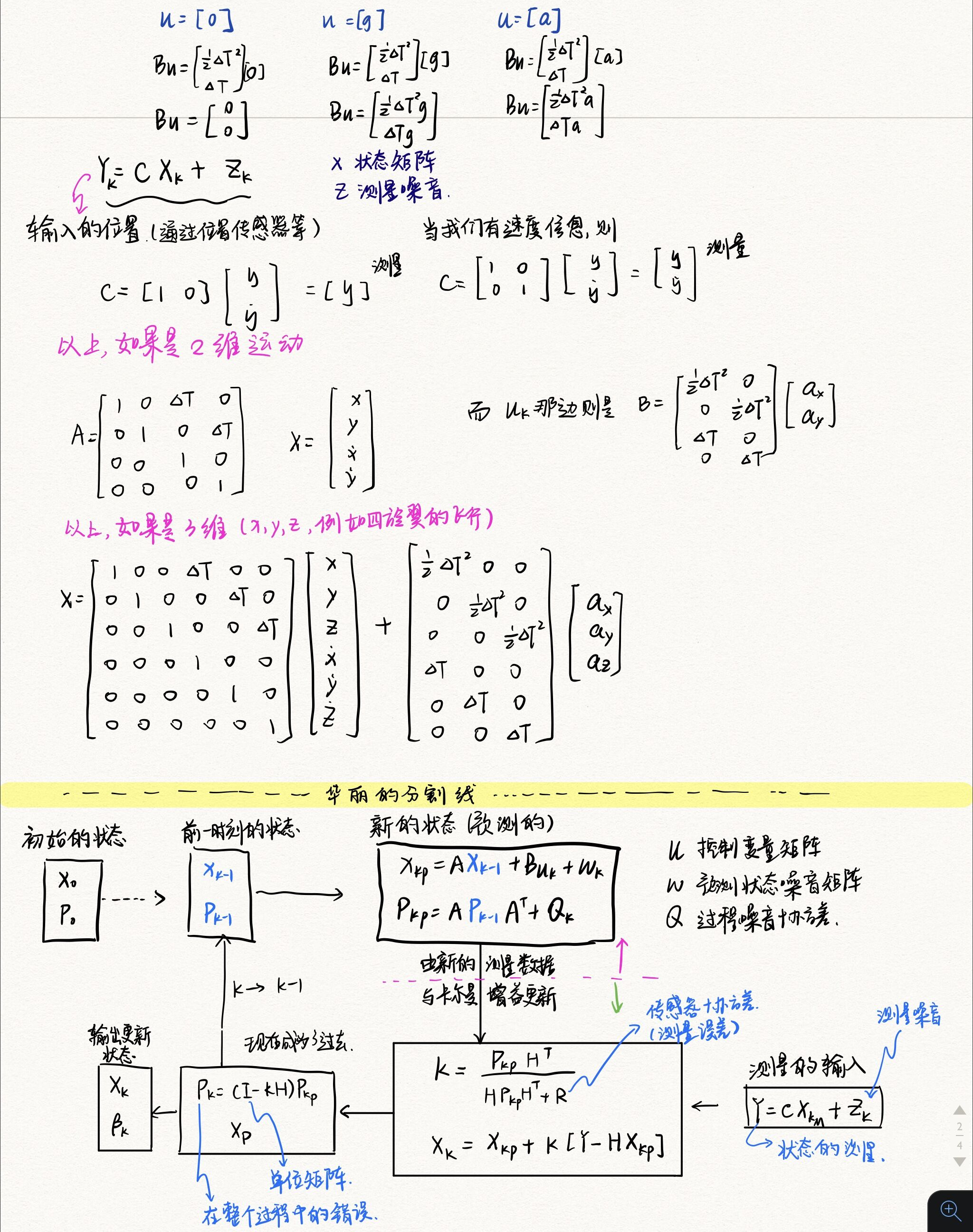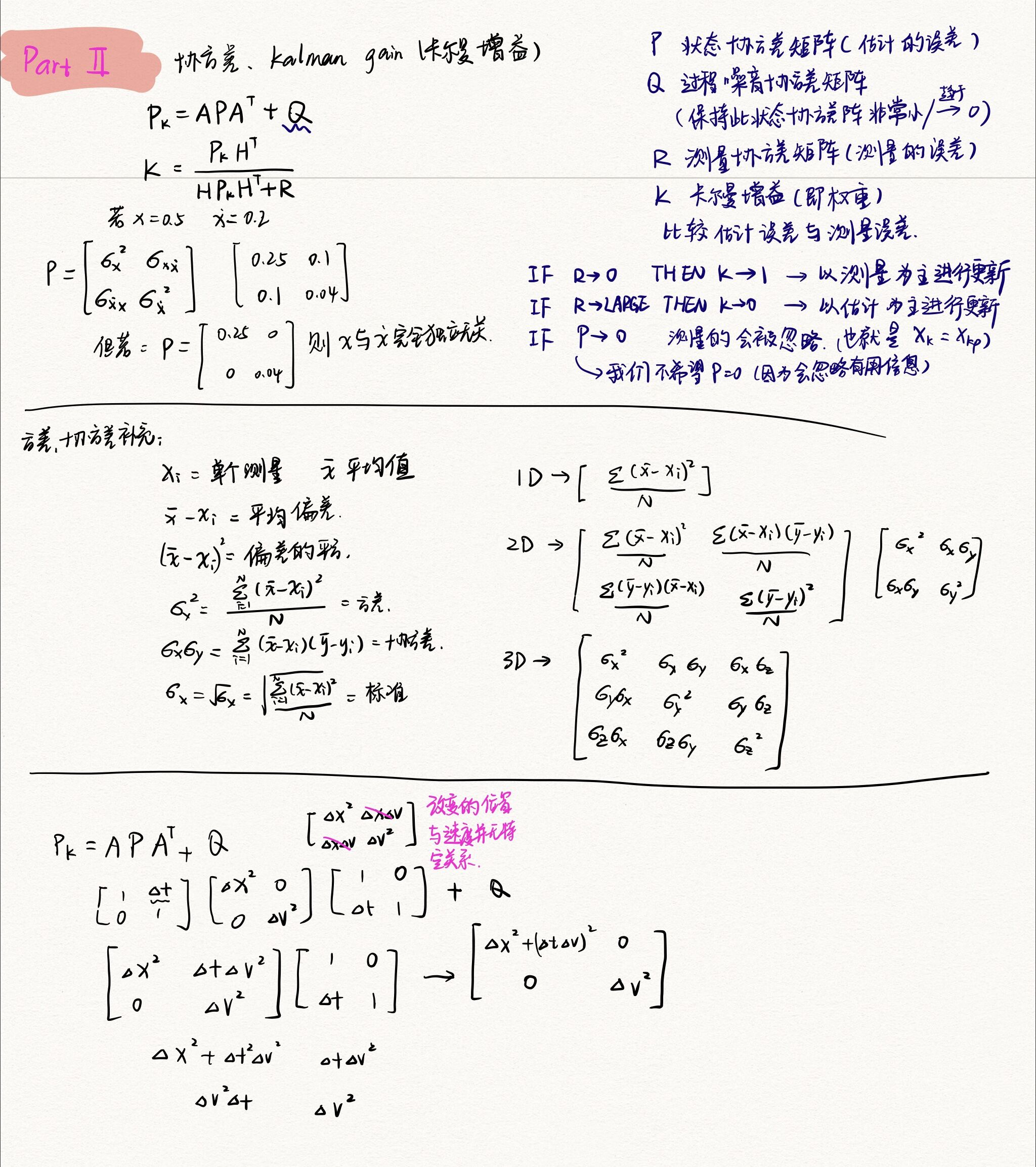emm 为什么后面都没记了呢，因为后面… 都在举那个上升气球的数值例子… 都是数字了，那么，不知道你们这么看能不能看懂哎… 希望大家都能看懂吧…（不枉费我对着看了一下午的视频）
首先，说一下笔记中的C和H是一样的，具体为什么，我看了很多人的解释说是：为了把卡尔曼的增益矩阵形式变成与H/C一样的… emm，大概就是这么个意思。
那么接下来？nonono 不是代码，是讨论区的题目TIPS：WEEK 2 讨论区
Part 1. Multi-dimensional Kalman Filter in general case.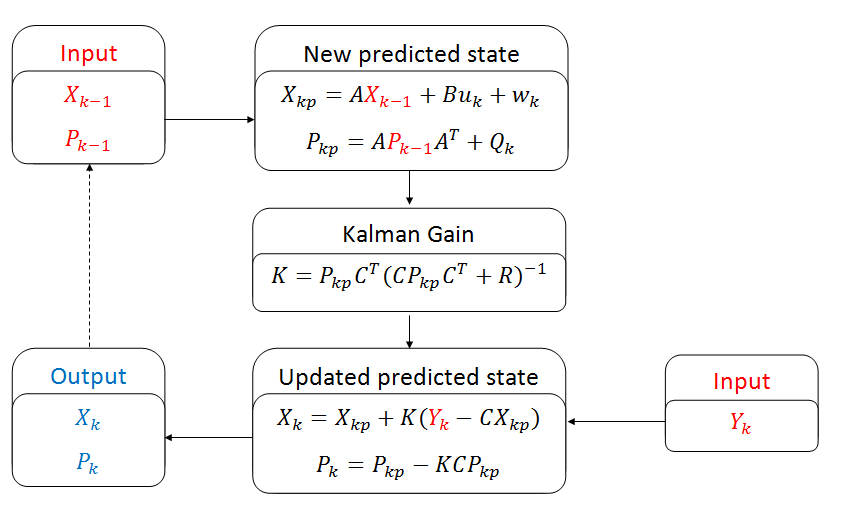这里面的字母意思如下：（和我上面的笔记可能字母有点不一样，正好也再理解理解吧~）

X is state (for ex., 4x1 vector: {x ;y; vx ;vy} )
P is state covariance matrix (for ex., 4x4 matrix)
A is transition matrix for velocity term (for ex., 4x4 matrix)
B is transition matrix for acceleration term (for ex., 4x2 matrix)
u is acceleration matrix (for ex., 2x1 matrix)
w is matrix representing noise in the process (for ex., 4x1 matrix)
Q is system noise covariance matrix (for ex., 4x4 matrix)
K is Kalman Gain (for ex., 4x2 matrix)
C is observation matrix (for ex. 2x4 matrix)
R is measurement noise covaiance matrix (for ex., 2x2 matrix)
Y is measurement matrix (for ex., 2x1 matrix: {z_x; z_y})

Part 2. Multi-dimensional Kalman Filter for this assignment case.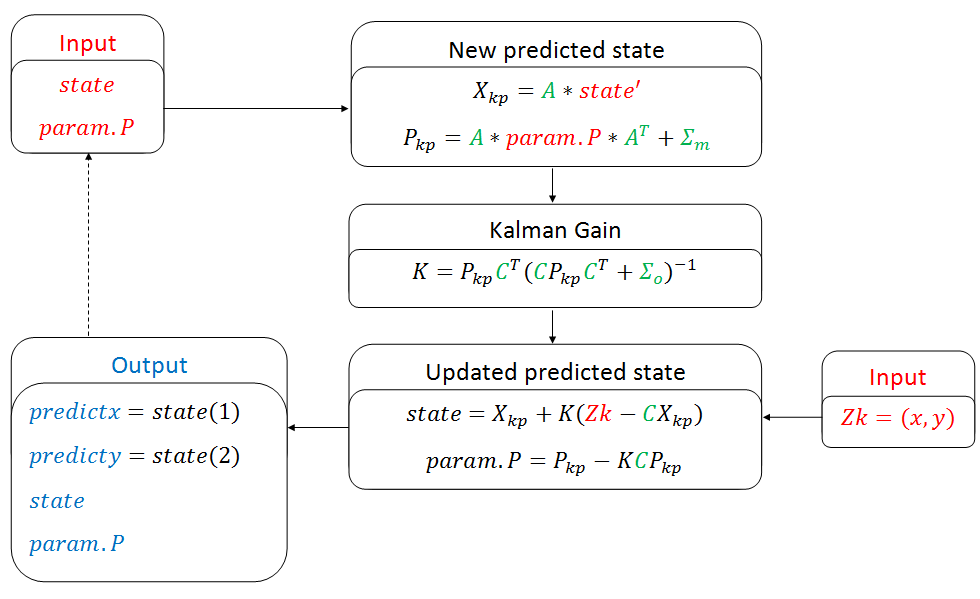Part 3. Code skeleton.
这里是代码骨架，没有答案的哦，可以先自己尝试一下，用上面的知识和这个框架先写一写
function [ predictx, predicty, state, param ] = kalmanFilter( t, x, y, state, param, previous_t )
%UNTITLED Summary of this function goes here
%   Four dimensional state: position_x, position_y, velocity_x, velocity_y

%% Place parameters like covarainces, etc. here:
dt = 0.033;

% Noise covariance matrices
Sigma_m =
Sigma_o =

% Transition matrix
A =

% Observation matrix
C =

% Check if the first time running this function
if previous_t<0
state = [x, y, 0, 0];
param.P = Sigma_m;
predictx = x;
predicty = y;
return;
end

% Predicted state & covariance matrix
Xkp =
Pkp =

% Kalman gain
K =

% Z measrement
Z =

% Update covariance matrix
param.P =

% Update state
state =

% Prediction
predictx = state(1);
predicty = state(2);

end

emm 说了那么多，终于到答案和注意事项的时候了，其实也不是… 答案，大家各有想法不用唯一化~
1.你问我… 噪音协方差你咋知道的？
emm 我不知道… 但是听视频说，协方差别=0，放个比较小的就行（PS 实际上呢，你得看你传感器的性质而定哈）
2.其他的代码中的，A、C、Xkp、Pkp一系列的都在上面的笔记和讨论区的图里了。
function [ predictx, predicty, state, param ] = kalmanFilter( t, x, y, state, param, previous_t )
%UNTITLED Summary of this function goes here
%   Four dimensional state: position_x, position_y, velocity_x, velocity_y

%% Place parameters like covarainces, etc. here:
dt = 0.033;
% Noise covariance matrices
Sigma_m = 0.01 * eye(4);
Sigma_o = 0.01 * eye(2);

% Transition matrix
A = [1 0 dt 0;
0 1 0 dt;
0 0 1 0;
0 0 0 1];

% Observation matrix
C = [1 0 0 0;
0 1 0 0];

% Check if the first time running this function
if previous_t<0
state = [x, y, 0, 0];
param.P = 0.001 * eye(4);
predictx = x;
predicty = y;
return;
end

% Predicted state & covariance matrix
Xkp = A*state';
Pkp = A*param.P*A'+Sigma_m;
R=Sigma_o;
% Kalman gain
K = Pkp*C'/(C*Pkp*C'+R);

% Y/Z measrement
Y = [x y]';

% Update covariance matrix
param.P = Pkp-K*C*Pkp;

% Update state
state = (Xkp+K*(Y-C*Xkp))';

% Prediction
predictx = state(1)+dt * state(3);
predicty = state(2)+dt * state(4);

end

所以… 现在大家应该没得问题了，PS最后的更新state记得转置（因为下一次你还得按以前格式输进来，不然又报错矩阵维度不一致了，以上，虽然这周的课不行，但是秉承着翻译的初衷，还是都翻译了…
最后，希望大家能真正看懂卡尔曼滤波的预测了（虽然我还是对那个C和H有点迷） 。走的时候留个赞，三连什么的最好了。
C和H那里搞懂了，又看了一遍其他视频的卡尔曼滤波的理解，C和H是一阶偏微分，也就是Jacobians矩阵，看什么时候有时间再开一个帖子专门整理一下这些天看过的卡尔曼滤波预测（这么一数下来，我似乎看了1、2、3、4个讲解视频，1、2个pdf文档，卡尔曼自己写的论文，emm 还拿MATLAB和Python实践了2遍… ）


展开全文• 本文厚颜承接 白色巧克力亦唯心的博文卡尔曼滤波 -- 从推导到应用（二） 写一些修正和理解总结
本文承接 白色巧克力亦唯心的博文卡尔曼滤波 -- 从推导到应用（二） 写一些修正和理解总结
刚刚步入机器人定位导航领域，内有感性理解，如有纰缪请指正，仅供交流。非常感谢白色巧克力亦唯心的总结与分享。精彩的博文，巨大的阅读量，对相关领域的蓬勃发展，将来可以说一句居功至伟。
卡尔曼滤波 -- 从推导到应用（一）公式推导细致入微。
卡尔曼滤波 -- 从推导到应用（二）例子承接（一），分析的很好，但有如下问题。
1、真实值并不是x，x是预测值。
真实值应该是：
xr=1/2*g*t.^2+Qn(int32(t/delta_t+1));%真实位置。其中数组Qn是每一个delta_t 的累计误差。每一次delta_t的噪声服从正态分布，均值为p，方差为q。
2、测量更新里测量的结果是真实值+测量噪声所以应该是：
z = xr +s+ r.*randn(1,N); % 测量时加入测量噪声。噪声R服从均值s,方差r的正态分布。
所以为了更真实地仿真滤波情景，代码修改后如下：

clc
clear all  ;
close all  ;

%% 初始化参数
delta_t=0.1;   %采样时间
t=0:delta_t:10;
N = length(t); % 序列的长度
sz = [2,N];    % 信号需开辟的内存空间大小  2行*N列  2:为状态向量的维数n
g=10;          %加速度值
p = 0;         %期望u
q = 10;      %方差sigma
s = 2;        %期望
r = 5;        %方差sigma
Q =[q 0;0 0]; %假设建立的模型  噪声方差叠加在位移上 大小为n*n方阵 n=状态向量的维数
R = r;    % 位置测量方差估计，可以改变它来看不同效果  m*m      m=z(i)的维数
%%
A=[1 delta_t;0 1];  % n*n
B=[1/2*delta_t^2;delta_t];
H=[1,0];            % m*n

n=size(Q);  %n为一个1*2的向量  Q为方阵
m=size(R);

% 分配空间
P=zeros(n);           % 后验方差估计  n*n
P(1) = 3.6603;

%%           构造系统误差数组Qn，下标n为每一时刻点的累计误差p+q*randn~Z(p,q)
Qn = zeros(1,N);
%%Statistics and Machine Learning Toolbox
Qn(1) = q*randn;
for i=2:1:N
Qn(i) = Qn(i-1) + q*randn;
end
%%

x=1/2*g*t.^2;      %预测位置
xr=1/2*g*t.^2+Qn(int32(t/delta_t+1));%真实位置
%z = x + sqrt(10).*randn(1,N); % 测量时加入测量白噪声
z = xr +s+ r.*randn(1,N); % 测量时加入测量白噪声                                     %%

xhat=zeros(sz);       % x的后验估计
xhatminus=zeros(sz);  % x的先验估计
Pminus=zeros(n);      % n*n
K=zeros(n(1),m(1));   % Kalman增益  n*m
I=eye(n);

%% 估计的初始值都为默认的0，即P=[0 0;0 0],xhat=0
for k = 9:N           %假设车子已经运动9个delta_T了，我们才开始估计
% 时间更新过程
xhatminus(:,k) = A*xhat(:,k-1)+B*g;
Pminus= A*P*A'+Q

% 测量更新过程
K = Pminus*H'*inv( H*Pminus*H'+R )
xhat(:,k) = xhatminus(:,k)+K*(z(k)-H*xhatminus(:,k));
P = (I-K*H)*Pminus
end
%%
figure(1)
plot(t,z);
hold on
plot(t,xhat(1,:),'r-')
plot(t,x(1,:),'k-');
hold on
plot(t,xr(1,:),'g-');
legend('含有噪声的测量', '后验估计', '独立预测值','真值');
xlabel('Iteration');


结果是这个样子的（每一次运行不一样，因为噪声是随机的）：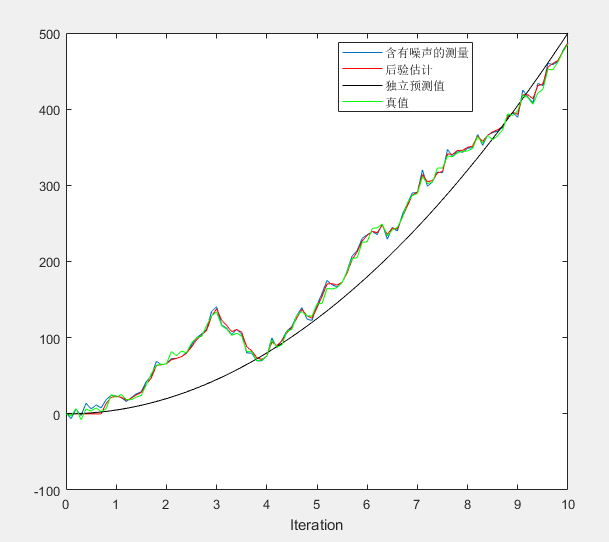我们说卡尔曼滤波的问题实际上是预测和测量我们更相信谁的问题。这样说很好理解，但这是一个静态的理解。

实际上卡尔曼滤波是一个动态的过程。
预测，有误差分布Q
测量，有误差分布R
真值 =预测-Q
真值 =测量-R
无论是预测还是测量，每一步都是
预测（测量-R）+Q
测量（预测-Q）
一定要理解：
每一波测量都在前一步的预测和测量的基础上，而不是孤立的测量。
每一步的预测是基于前一步的预测和测量，而不是孤立的预测。如matlab曲线中的独立预测值曲线。
每一步的测量测量的是真实值也就是测的是上一步的预测-Q，所以测量不是孤立的。所以我们看到后验曲线在测量曲线周围而不在孤立的预测值曲线周围。

下面回答一下文章的标题。

数学角度：（公式摘自白色巧克力亦唯心 卡尔曼滤波 -- 从推导到应用（一））
我们该相信谁？那么我们来求K吧。从贝叶斯估计的理念讲，权重，这一步就是表示这个权重，该相信谁多少。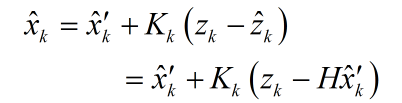怎么求K？求K使得估计值与真实值的最小均方差最小。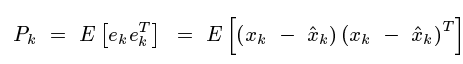详细推导见 白色巧克力亦唯心 卡尔曼滤波 -- 从推导到应用（一）。

一开始要假定一个P的初值，相当于我们一开始先假定该相信谁多少。后面的每一步迭代实际上就是根据由两个噪声分布得到的数据去计算K。最终K，P，Pminus都收敛了（在本模型下，其它未知模型请自行验证，感悟）。
这个收敛的值就是我们应该相信谁的一个权重。下面结合上面的代码看一下输出值：

设置 P(1) =10 ：

Pminus =    20     0     0     0K =    0.8000         0P =    4.0000         0         0         0Pminus =    14     0     0     0K =    0.7368         0P =    3.6842         0         0         0Pminus =   13.6842         0         0         0K =    0.7324         0P =    3.6620         0         0         0Pminus =   13.6620         0         0         0K =    0.7321         0P =    3.6604         0         0         0Pminus =   13.6604         0         0         0K =    0.7321         0P =    3.6603         0         0         0Pminus =   13.6603         0         0         0K =    0.7321         0P =    3.6603         0         0         0

一开始就设置p(1) =3.6603：
Pminus =   13.6603         0         0         0K =    0.7321         0P =    3.6603         0         0         0Pminus =   13.6603         0         0         0K =    0.7321         0P =    3.6603         0         0         0
从一开始就把收敛值带入那么从一开始就收敛。

小结：
按照我的理解（刚入职，工作原因暂未查询资料验证，仅供交流），如果预测的噪声分布Q和测量的噪声分布R确定，那么该相信谁更多，K从一开始就是客观存在的，是确定的。而预测更新和测量更新，其实是一个通过迭代去得到这个确定的K值的过程。 方法就是求K使得 每次迭代的 估计值与真实值的最小均方差最小。

那么既然该相信谁更多实际上是确定的，那么如果测量过程中出现高斯分布边缘的小概率的大误差卡尔曼滤波能否有效降低这个大的误差？
答案应该是否定的。 我们最终将以同样的收敛的K的权重去相信两者，所以误差是与测量误差成线性关系的。
更相信谁与单个样本点无关，只与系统噪声和测量噪声的分布有关。
所以，卡尔曼滤波可以通过预测对测量进行优化，但是对于大的测量（如大的距离的匹配失准）是无能为力的。所以我们可以用卡尔曼滤波做优化，但是对于可能出现的大的误差或者说错误，需要想别的办法特殊处理。


展开全文• %在二维空间,假设运动物体的一组运动数据,用卡尔曼滤波方法进行处理,两点起始法%实验室的博客clear;% 1.1.实际和测量的运动数据,线性运动% rx = 0:0.1:10;%x方向实际运动轨迹% ry = 2*rx;%y方向实际运动轨迹% 1.2....
%在二维空间,假设运动物体的一组运动数据,用卡尔曼滤波方法进行处理,两点起始法%实验室的博客clear;% 1.1.实际和测量的运动数据,线性运动% rx = 0:0.1:10;%x方向实际运动轨迹% ry = 2*rx;%y方向实际运动轨迹% 1.2.实际和测量的运动数据,运动轨迹为抛物线rx = 0:0.1:10;%x方向实际运动轨迹ry = rx.^2;%y方向实际运动轨迹% 1.3.实际和测量的运动数据,运动轨迹为弧线% theta =0:0.01:(pi/2);% rx =10*cos(theta); %x方向实际运动轨迹% ry =10*sin(theta); %y方向实际运动轨迹sigma = 0.1;%x,y方向的测量噪声,假设两方向测量噪声相同Z = [rx; ry] +0.1*wgn(2, length(rx), 5); %测量的运动轨迹% Z = [rx; ry] +sqrt(sigma) * randn(2, length(rx)); %测量的运动轨迹T = 0.1;%测量周期time =(length(rx)-1) * T;% 2.1参数设置,只有位置和速度信息% X0 = [rx(2);ry(2); (rx(2)-rx(1))/T; (ry(2)-ry(1))/T]; %初始位置和速度状态% P0 = [1 0 0 0;0 1 0 0; 0 0 100 0; 0 0 0 100]; %初始协方差矩阵% F = [1 0 T 0; 01 0 T; 0 0 1 0; 0 0 0 1]; %状态矩阵% H = [1 0 0 0; 01 0 0]; %观测矩阵% 2.2参数设置,包括位置、速度和加速度信息X0 = [rx(2);ry(2); (rx(2)-rx(1))/T; (ry(2)-ry(1))/T; (rx(2)-rx(1))/T^2;(ry(2)-ry(1))/T^2]; %初始位置,速度和加速度状态P0 = [1 0 0 0 00; 0 1 0 0 0 0; 0 0 10 0 0 0; ...0 0 0 10 0 0; 0 00 0 10 0; 0 0 0 0 0 10]; %初始协方差矩阵F = [1 0 T 00.5*T^2 0; 0 1 0 T 0 0.5*T^2; 0 0 1 0 T 0;...0 0 0 1 0 T; 0 00 0 1 0; 0 0 0 0 0 1]; %状态矩阵H = [1 0 0 0 0 0;0 1 0 0 0 0]; %观测矩阵Q = 1e-3*eye(6); %过程噪声 该值越大 滤波结果越接近测量值R = [sigma 0; 0sigma]; %测量噪声矩阵X =zeros(length(X0),length(rx));X(:, 1) = [rx(1);ry(1); zeros(length(X0)-2, 1)];X(:, 2) =X0;for i =3:length(rx)%预测Xk = F * X0;%预测状态Pk = F * P0 * F'+ Q; %预测估计协方差矩阵%更新yk = Z(:, i) - H* Xk; %测量余量Sk = H * Pk * H'+ R; %测量余量协方差Kk = Pk * H' /Sk; %最优卡尔曼增益X0 = Xk + Kk *yk; %更新的状态估计X(:, i) =X0;P0 =(eye(length(X0)) - Kk * H) * Pk; %更新的协方差估计endfigure(1)plot(rx,ry,'r',Z(1,:),Z(2,:),'g', X(1,:),X(2,:),'b');xlabel('x','fontsize',10);ylabel('y','fontsize',10); grid on;% axis([0 12 012]);axis([0 10 0100]);legend('实际运动轨迹','测量运动轨迹','滤波运动轨迹');figure(2)t =0:T:time;subplot(2,1,1);plot(t,X(3,:));title('x方向速度');xlabel('t','fontsize',10);ylabel('Vx','fontsize',10);grid on;subplot(2,1,2);plot(t,X(4,:));title('y方向速度');xlabel('t','fontsize',10);ylabel('Vy','fontsize',10);grid on;figure(3)t =0:T:T*(length(rx)-1);subplot(2,1,1);plot(t,X(5,:));title('x方向加速度');xlabel('t','fontsize',10);ylabel('ax','fontsize',10);grid on;subplot(2,1,2);plot(t,X(6,:));title('y方向加速度');xlabel('t','fontsize',10);ylabel('ay','fontsize',10);grid on;如果你有所收获，欢迎用微信扫一扫进行打赏，赏金随意。展开全文• %%%%%%%%%%%%%%%%%%%%%%%%%%%%%%%%%%%%%%%%%%%%%%%%%%%%%%%%%%%%%%%%%%%%%%%%%%%%%%%%%%%%%%%%%%%%%%%%%%%基于Kalman滤波算法的自适应AR模型%%%%%%%%%%%%%%%%%%%%%%%%优点：算法收敛速度快；缺点：算法较复杂。clc;...
%%%%%%%%%%%%%%%%%%%%%%%%%%%%%%%%%%%%%%%%%%%%%%%%%%%%%%%%%%%%%%%%%%%%%%%%%%%%%%%%%%%%%%%%%%%%%%%%%%%基于Kalman滤波算法的自适应AR模型%%%%%%%%%%%%%%%%%%%%%%%%优点：算法收敛速度快；缺点：算法较复杂。clc;clear all;close all;load data3;z=A3;n=length(z);N=8108;M=18;%预报步数M1=30;%最大阶数n=18;%预报步数，调整n值可得对应步数下的性能指标值c=1;%c为小的正数q=1;avrg=sum(z(1:N))/N;xg=zeros(N+M,1);s=zeros(M,1);a=zeros(M1,1);for i=1:N;xg(i)=z(i)-avrg;end%定阶A=zeros(M1,M1);%对应每列保存1:p(AIC准则下的阶数)对应的参数faifor p=1:M1;fai=zeros(p,N);v1=randn(1,p)*0.001;v2=randn(1,p)*0.001;I=eye(p,p);k=repmat(I,[1,1,N+1]);%k(t,t-1)=k(:,:,t);g=zeros(p,N);k_k=repmat(I,[1,1,N+1]);fai(:,p)=zeros(p,1);k(:,:,p+1)=c*I;xi=zeros(p,N+M);arfa=zeros(N,1);for i=p:N;xi(:,i)=xg(i:-1:i-p+1);end%%%预先假设v1、v2的方差,v2方差为(0.001~0.01)*(x(t)的方差)%Jmin=0.001*var(z(p+1:N)); %这里消除认为设定for i=p:N-1;%g(:,i+1)=k(:,:,i+1)*xi(:,i)*inv(xi(:,i)'*k(:,:,i+1)*xi(:,i)+Jmin);g(:,i+1)=k(:,:,i+1)*xi(:,i)*inv(xi(:,i)'*k(:,:,i+1)*xi(:,i));arfa(i+1)=xg(i+1)-xi(:,i)'*fai(:,i);fai(:,i+1)=fai(:,i)+g(:,i+1)*arfa(i+1);k_k(:,:,i+1)=k(:,:,i+1)-g(:,i+1)*xi(:,i)'*k(:,:,i+1);k(:,:,i+2)=k_k(:,:,i+1)+10^-2*I;endfai(:,N);for i=1:p;A(i,p)=fai(i,N);endx_yb=zeros(N-p,1);x_y=zeros(N-p,1);for i=p+1:N;x_y(i)=fai(:,N)'*xi(:,i-1);endfor i=p+1:N;x_yb(i)=x_y(i)+avrg;endg=zeros(N-p,1);for i=p+1:N;g(i)=z(i)-x_yb(i);endh=sum(g.^2);%a(p)=log(h/(N-2*p-1))+2*p/N;a(p)=log(h/(N-2*p-1))+2*(p+1)*log(N)/N;end[C,p]=min(a)Fai=zeros(p,1);for i=1:p;Fai(i)=A(i,p);end%预报x_y1=zeros(N+M,1);xg(N+1)=Fai'*xg(N:-1:N-p+1);for i=N+1:N+M;xg(i+1)=Fai'*xg(i:-1:i-p+1);endfor i=1:N+M;x_y1(i)=xg(i)+avrg;end%绘图figure(1);k=N-10:N+M;k1=N-10:N+18;plot(k1,z(k1),'r*-');hold on;k=N:N+M;plot(k,x_y1(k),'b*-');xlabel('t步长');ylabel('角');legend('GBPUSD磅美真实值','预报值');%性能指标%y=max(abs(x(N+1:N+n)));%z=zeros(n,1);%for i=N+1:N+n%z(i)=x(i)-x_y1(i);%end%S=sum(z.^2);%S1=sqrt(S/n);%yit=S1/y
展开全文• 卡尔曼滤波利用目标的动态信息与观测结果相结合，抑制噪声的影响，从而获得一个关于目标位置更准确的估计，这个估计可以是对当前目标位置的估计(滤波)，也可以是对于将来位置的估计(预测)，也可以是对过去位置的估计...信号处理 自适应卡尔曼
• 我正在学习卡尔曼滤波，目的是预测弹道。现在，我可以跟踪球了。在我第一次使用预测和kalman滤波器进行实际尝试时，我使用了一个绘制直线的示例，如下所示：以下是完整代码：import cv2import numpy as npimport ...
• 1. 理论 最近学习卡尔曼滤波，由于卡尔曼滤波的公式...2. 无人机轨迹预测与仿真 （卡尔曼滤波） 2.1 卡尔曼滤波理解 总体来说仿真卡尔曼滤波就是在已知状态方程的情况下得到各矩阵的值，先算出加上高斯噪声的测量值，得
• 所有滤波问题其实都是求感兴趣的状态的后验概率分布，只是由于针对特定条件的不同，可通过求解析解获得后验...是通过观测信息及状态转移及观测模型对状态进行光滑、滤波预测的方法。而KF、EKF及UKF的滤波问题都...
• 在任何含有不确定信息的动态系统中使用卡尔曼滤波，对系统下一步的走向做出有根据的预测，即使伴随着各种干扰，卡尔曼滤波总是能指出真实发生的情况。 一、卡尔曼的五个基本公式 二、理解要点 1.所谓卡尔曼滤波...
• 预测步骤如图1所示：图1 卡尔曼滤波原理流程图假设我们能够得到被测物体的位置和速度的测量值，在已知上一时刻的最优估计值以及它的协方差矩阵的条件下(初始值可以随意取，但协方差矩阵应为非0矩阵)，则有，，即： ....
• 卡尔曼滤波算法分为两步：预测和更新 预测：根据上一时刻（k-1时刻）的后验估计值来估计当前时刻的状态，得到k时刻的先验估计值。（时间更新方程或预测方程） 更新：使用当前时刻的测量值来更正预测阶段估计值。...
• 卡尔曼滤波是一种结合预测（先验分布）和测量更新（似然）的状态估计算法。 预测模块就是对物体的运动建立运动模型，本文栗子中的行人状态估计我们采用恒速度模型（CV），通过对上一时刻的最优估计进行运动模型转换...
• 卡尔曼滤波预测轨迹程序，Matlab程序可以帮助你学习卡尔曼滤波
• 无迹卡尔曼滤波与扩展卡尔曼纳滤波的对比，程序可改，主要是针对扩展卡尔曼纳滤波与扩展卡尔曼滤波预测性能做一个比较，程序可用，并且可以按照自己的函数进行更改
• 依旧是Python课程 _(:з」∠)_ 期末需要完成小组作业，选题内容主要是用卡尔曼滤波来实现交通参数的预测。已有数据包括：历时7天的上下匝道线圈数据，匝道口上下游的数据。数据采集点及数据结构如下图所示。数据结构...
• ）直观表示卡尔曼滤波干了啥，其实就是用贝叶斯，两个分布乘起来，得到的后验分布方差明显要小问题背景也很简洁，就是我们对于一个状态量x，例如位移、速度，我们可以通过初始条件直接给出基于物理学定律的预测。......